# Olympiad Test: Algebraic Expressions And Their Identities - 1

## 20 Questions MCQ Test Mathematical Olympiad Class 8 | Olympiad Test: Algebraic Expressions And Their Identities - 1

Description
Attempt Olympiad Test: Algebraic Expressions And Their Identities - 1 | 20 questions in 40 minutes | Mock test for Class 8 preparation | Free important questions MCQ to study Mathematical Olympiad Class 8 for Class 8 Exam | Download free PDF with solutions
QUESTION: 1

### What are the factors of the expression ab – a - b + 1?

Solution:

ab – a – b + 1
= a(b – 1) – 1 (b – 1) = (b – 1) (a – 1)

QUESTION: 2

### If (2x - 3) is a factor of 6x2 – 7x – 3, then what is the other factor?

Solution:

6x2 – 7x – 3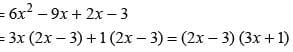QUESTION: 3

### What are the factors of (g + h)2 - 4gh ?

Solution: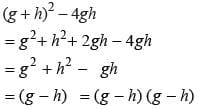QUESTION: 4

If one of the factor of 25(x + y)2 – 36 (x – 2y)2 is (17y – x), then what is the other factor?

Solution:

25 (x + y)2 – 36 (x – 2y)2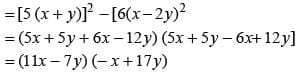QUESTION: 5

Find the value of (a + 1) (a – 1) (a2 + 1).

Solution: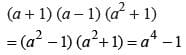QUESTION: 6

If x + y = 12 and xy = 14 then what is the value of x2 + y2?

Solution:

(x + y)2 = x2 + y2 + 2xy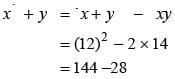= 116

QUESTION: 7

​If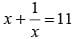then what is the value of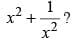Solution: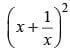=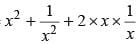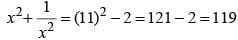QUESTION: 8

If x + y = 10 and xy = 9, what is the value of x2 – y2?

Solution:

( x + y) 2 = x2 + y2+ 2xy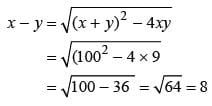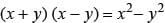∴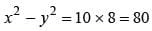QUESTION: 9

If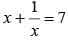then find the value of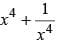.

Solution: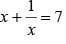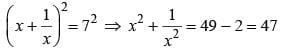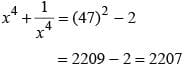QUESTION: 10

The perimeter of a triangle is 6m2 – 4m + 9 and two of the sides are m2 – 2m + 1 and 2m2 + 3m + 5. What is the third side?

Solution:

Third side = 6m2 – 4m + 9 – (m2 – 2m + 1 + 2m2 + 3m + 5)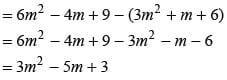QUESTION: 11

What is the remainder when 7 + 15x – 13x2 + 5x3 is divided by 4 – 3x + x2?

Solution: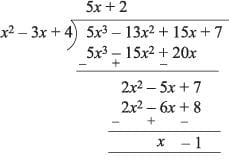Clearly (x - 1) is remainder.

QUESTION: 12

What is the quotient if x4 – 2x3 + 2x2 + x + 4 is divided by x2 + x + 1?

Solution: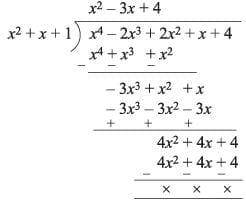∴ Quotient = x2 - 3x + 4

QUESTION: 13

What is the quotient if 5x3 – 4x2 + 3x + 18 is divided by 3 – 2x + x2?

Solution: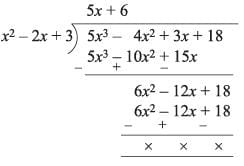QUESTION: 14

​If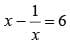then what is the value of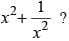Solution: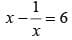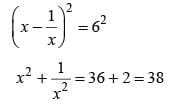QUESTION: 15

8a2 b3 ÷ (-2ab)?

Solution: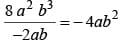QUESTION: 16

What is the value of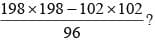Solution: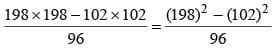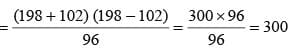QUESTION: 17

What is the numerical coefficient in the product of 2abc, - 16a2bc and 3ab2c2?

Solution:

(2abc) (- 16 a2 bc) (3a b2 c2)
= - 96 a4 b4 c3

QUESTION: 18

​If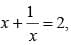what is the value of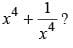?

Solution: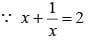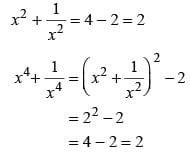QUESTION: 19

What is the value of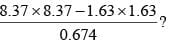Solution: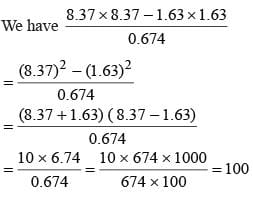QUESTION: 20

What is the remainder when 6x2 – 11x + 15 is divided by 2x – 5?

Solution: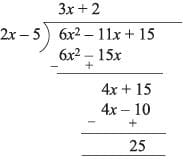Use Code STAYHOME200 and get INR 200 additional OFF Use Coupon Code